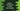# Introduction to yield in Python with example## Introduction to yield in Python with example:

In this post, we will learn what is yield and how to use yield in Python with examples.

yield statement is similar to the return statement we used to return values from a function. But, there is a difference. This statement doesn’t return a value. It returns a generator object. What is a generator object and how to read the data from it? Let’s learn:

### What is yield keyword:

yield keyword returns some value from a function in Python, but it doesn’t kills the state of local variables of the function. That means, it will keep executing the function after yield is called.

This is the difference between yield and return. If we use return with a function, it returns the value and then it exits from the function. But, if we use a yield, it will not exit from a function. We can use multiple yield statements in a function.

### Generator function:

A function is called a generator function if it contains at least one yield statement. These types of functions can keep generating values. It doesn’t return a single value, but returns an generator object. The caller function can loop over the values in this object. We can use list(), a for loop or next() to read the contents of a generator object.

### Syntax of yield:

yield is defined as like below:

``yield expression``

yield returns a generator object. As explained before, we need to iterate throught this object to read the values.

### Example of yield:

Let’s take a small example of yield.

``````def getMessage():
yield 'Hello World'

print(getMessage())``````

If you run this code, it will print:

``<generator object getMessage at 0x1050ea740>``

As you can see here, it returns a generator object. To read the data, we have to itrate over it.

``````def getMessage():
yield 'Hello World'

for msg in getMessage():
print(msg)``````

It will print:

``Hello World``

Now, if we use multiple yield, it will print them all:

``````def getMessage():
yield 'Hello World'
yield 'Hello Universe'

for msg in getMessage():
print(msg)``````

It will print:

``````Hello World
Hello Universe``````

If we use return, we can get only one return value from a function. But, it we use yield, we can have multiple return values.

## How to read the values from a generator object:

We need to iterate over the generator object to read its contents. We can use a for loop or we can use the next() or list() method to read the contents.

Let me show you how to do that for each:

### Example with a for loop:

Let’s have a look at the below program:

``````def getSquares():
for i in range(0, 10):
yield i * i

for num in getSquares():
print(num)``````

In this example, getSquares method uses yield to return squares of numbers from 0 to 9. We are using a for loop to iterate through the numbers of the return values.

If you run this program, it will print:

``````0
1
4
9
16
25
36
49
64
81``````

### Example with next():

We can use next() method to read the contents of an iterator. It returns the next value from an iterator. It raises StopIteration exception when the iterator is exhausted.

``````def getSquares():
for i in range(0, 5):
yield i * i

squares = getSquares()

print(next(squares))
print(next(squares))
print(next(squares))
print(next(squares))
print(next(squares))
print(next(squares))``````

In this program, we are using yield from 0 to 4. If you run this, it will print:

``````0
1
4
9
16
Traceback (most recent call last):
File "example.py", line 12, in <module>
print(next(squares))
StopIteration``````

As you can see here, it raised StopIteration once it is exhausted.

### Example with list():

list() function can be used to create a list from an iterable. We can use it with a generator object to get all values of the generator in a list. For example,

``````def getSquares():
for i in range(0, 10):
yield i * i

squares = list(getSquares())

print(squares)``````

It will print:

``[0, 1, 4, 9, 16, 25, 36, 49, 64, 81]``

## Generators can’t be used for multiple times:

We can’t use a generator object more than once. It will be empty if we try to use it. For example:

``````def getSquares():
for i in range(0, 5):
yield i * i

squares = getSquares()

print(f'Squares: {list(squares)}')
print(f'Squares: {list(squares)}')``````

It will print:

``````Squares: [0, 1, 4, 9, 16]
Squares: []``````

As you can see here, it printed an empty list for the second time.

## Example of yield to print the Fibonacci series:

Let’s take an example of yield. This program will print the Fibonacci series.

``````def get_fibonacci(limit):
current_value = 0
next_value = 1

for i in range(0, limit):
yield current_value
sum = current_value + next_value
current_value = next_value
next_value = sum

for i in get_fibonacci(10):
print(i, end=' ')``````

Here,

• get_fibonacci function uses yield to return the fibonacci series values.
• The for loop runs for limit number of times.
• Inside the loop, we are using yield to generate current_value, which is the current value of the series.

If you run this, it will print the below output:

``0 1 1 2 3 5 8 13 21 34``